# Minimum Phase and All Pass Systems

### Topics Covered:

1. Minimum Phase System
2. All Pass System
3. System Function Factorization
4. Implications of the Factorization / Properties of Minimum Phase System
5. Factorization Algorithm
6. Magnitude Square Factorization

### Minimum Phase Systems:

A system function H(z) is said to be a minimum phase system if all of its poles and zeros are within the unit circle. Consider a causal and stable LTI system with a difference equation representation of the form: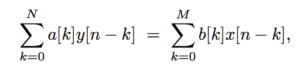the system function H(z) of this LTI system takes the form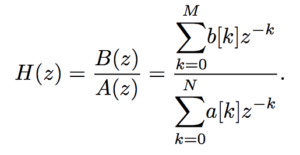In pole-zero format, this system function is given by: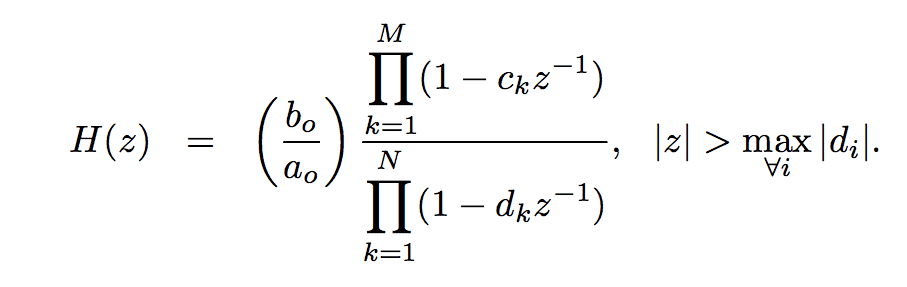The minimum phase condition on the system function can then be translated into the pole-zero parameters as: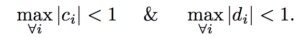The implication of this statement is that inverse system with system function Hi(z) also has its poles and zeros within the unit circle, i.e., Hi(z) is also a minimum phase system: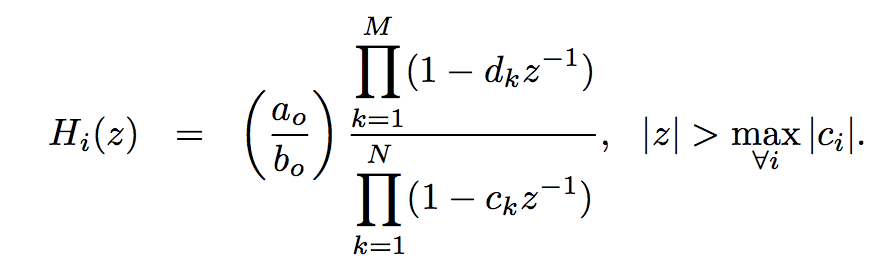This means that the minimum-phase system is a system where both the system and its inverse are causal and stable.

### All Pass Systems:

An allpass system is a system whose frequency response magnitude is constant for all frequencies, i.e.,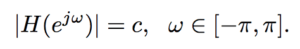The general form of an implementable allpass system is: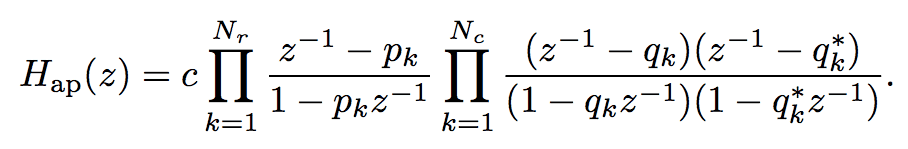Specifically let us look at the causal and stable LTI system with system function H(z) given by: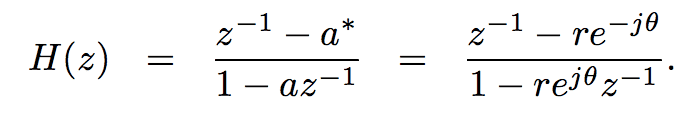The corresponding frequency response for this system is: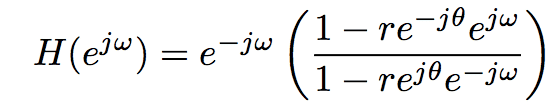The magnitude response of this system is given by: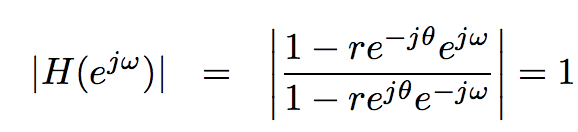Consequently, this system is an all-pass system of first order. The phase response of this system is given by: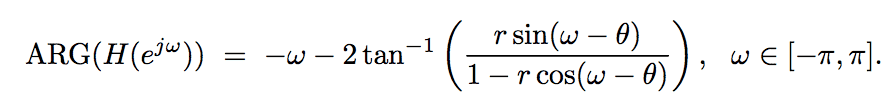The group delay of this system obtained after taking the derivative of the expression above is given by: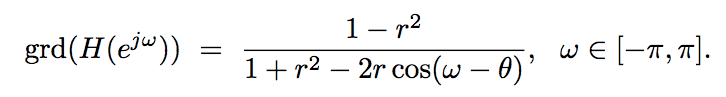From the expression above we can see that the allpass system is a positive group delay system, i.e.,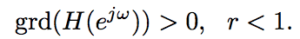It is easily seen that this implies that the continuous phase is negative over ω ∈ [0, π], i.e.,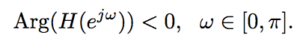### System Function Factorization:

Consider a stable and causal LTI system with system function H(z). Since the system is a stable system the poles of the system function are required to be inside the unit circle (UC). The zeroes are, however, free to wander outside. Let us assume that there are q zeroes of H(z) that are outside the UC. Then the system function H(z) can be rewritten as: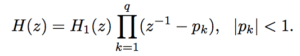Specifically, the system function H1(z) is a minimum phase system function because it has all its poles and zeroes inside the UC. Rewriting this expression to include the allpass factor we obtain: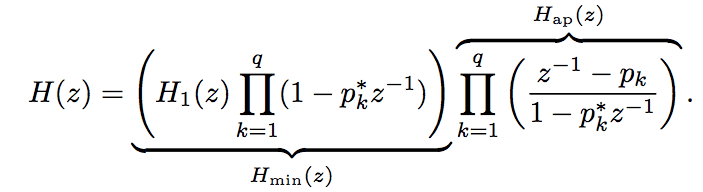This implies that system function H(z) can then be factorized into two parts as: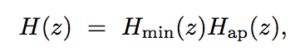where Hmin(z) is defined as the minimum phase component of H(z) and Hap(z) is defined as the allpass part or component of H(z).

### Implications of Factorization / Properties of minimum phase system:

In terms of the magnitude response |H(e)| this factorization implies that: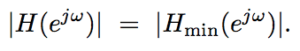In other words, the magnitude of the frequency response of the system and the magnitude of the frequency response of the minimum phase part are identical. In terms of the phase response, this factorization implies that: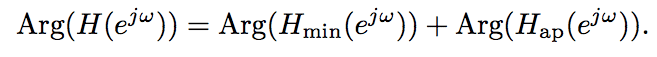This means that the minimum phase system is also a minimum phase lag system. In terms of group delay response this factorization implies: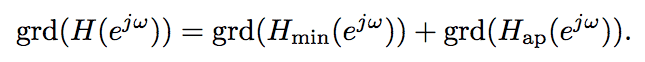Using the positive group delay property of the allpass part we can infer that the minimum phase system is also a minimum group delay system.

#### Factorization Algorithm:

The algorithm for obtaining the components is :

1. Assign the poles and the zeroes of H(z) inside the UC to the minimum phase part Hmin(z).
2. Reflect the zeroes of H(z) that are outside the UC to their conjugate reciprocal locations.
3. The allpass part will be composed of the zeroes that lie outside the UC and the poles at the complex reciprocal locations needed to compensate for the reflection of the zeroes to the complex reciprocal locations.

### Magnitude Square Factorization:

Consider a system function C(z) that is positive, real and analytic on the UC. Since the system function C(z) is positive, real and analytic on the UC, the corresponding frequency response can be expressed in the form: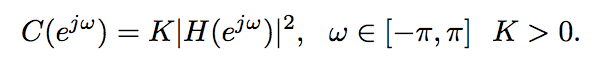The corresponding quantity system function in the Z-domain is: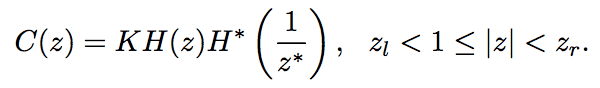This means that the poles and zeroes of C(z) come in conjugate reciprocal pairs. We can then split the poles and zeroes into two groups, one group inside the UC and the other group outside the UC as described by: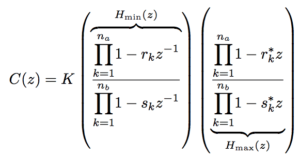Then the system function C(z) can be factorized in the form: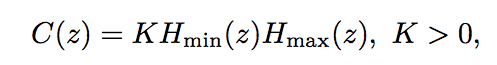where Hmin(z) is the minimum phase part or component of C(z) and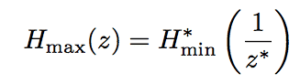is the maximum phase part of C(z). The corresponding in the time domain is: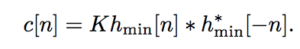This site uses Akismet to reduce spam. Learn how your comment data is processed.

error: